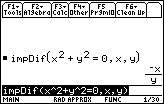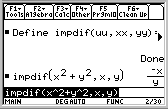# Knowledge Base

## Solution 12059: Implicit Partial Differentiation on the TI-89 Family, TI-92 Family, or Voyage™ 200 Graphing Calculators.

### How do I perform implicit partial differentiation on the TI-89 family, TI-92 family, or Voyage 200 graphing calculators?

The latest versions of the Voyage 200 and TI-89 Titanium operating system contain a impdif ( command.

This command should be used when computing a implicit partial differentiation.

To access the command, press [F3] [Up Arrow] [Enter] on the homescreen.

For example, to solve the equation x^2+y^2=0, enter impdif(x^2+y^2=0, x, y) and press [ENTER].If you are using the TI-89, the impdif( command is not built in the operating system. Implicit partial differentiation can be defined using the define command on the unit. Below is a first derivative partial differentiating function based on a more general function found in Derive™.

define impdif(uu, xx, yy)=-d(uu,xx)/d(uu,yy)

Where uu is an expression that equals 0, and d() is the derivative function. The variables xx and yy are used to avoid a circular definition error that will occur when you differentiate a function of x and y.

The function:

impdif(x^2+y^2,x,y)

Will return -x/y, which is the reduced form of -2x/(2y)Please see the TI-89 Titanium / Voyage 200 guidebook for additional information.